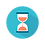From: Bayesian Models for Astrophysical Data, Cambridge Univ. Press

(c) 2017,  Joseph M. Hilbe, Rafael S. de Souza and Emille E. O. Ishida

you are kindly asked to include the complete citation if you used this material in a publication

Code 10.21 Lognormal–logit hurdle model, in Python using Stan, for assessing the relationship between dark halo mass and stellar mass

==================================================================================

import numpy as np
import pandas as pd
import pystan
import statsmodels.api as sm

# Data
path_to_data = ()

# prepare data for Stan
y = np.array([np.arcsinh(10**10*item) for item in data_frame['Mstar']])
x = np.array([np.log10(item) for item in data_frame['Mdm']])

data = {}
data['Y'] = y
data['Kc'] = data['Xc'].shape
data['Kb'] = data['Xb'].shape
data['N'] = data['Xc'].shape

# Fit
stan_code="""
data{
int<lower=0> N;                                      # number of data points
int<lower=0> Kc;                                    # number of coefficients
int<lower=0> Kb;
matrix[N,Kb] Xb;                                   # dark matter halo mass
matrix[N,Kc] Xc;
real<lower=0> Y[N];                             # stellar mass
}
parameters{
vector[Kc] beta;
vector[Kb] gamma;
real<lower=0> sigmaLN;
}
model{
vector[N] mu;
vector[N] Pi;

mu = Xc * beta;
for (i in 1:N) Pi[i] = inv_logit(Xb[i] * gamma);

# priors and likelihood
for (i in 1:Kc) beta[i] ~ normal(0, 100);
for (i in 1:Kb) gamma[i] ~ normal(0, 100);
sigmaLN ~ gamma(0.001, 0.001);

for (i in 1:N) {
(Y[i] == 0) ~ bernoulli(Pi[i]);
if (Y[i] > 0) Y[i] ~ lognormal(mu[i], sigmaLN);
}
}
"""

# Run mcmc
fit = pystan.stan(model_code=stan_code, data=data, iter=15000, chains=3,
warmup=5000, thin=1, n_jobs=3)

# Output
print(fit)

==================================================================================Output on screen:

Inference for Stan model: anon_model_c43c9b3fa0946fb161b5870b7e207048.
3 chains, each with iter=15000; warmup=5000; thin=1;
post-warmup draws per chain=10000, total post-warmup draws=30000.

mean      se_mean         sd       2.5%         25%      50%        75%      97.5%     n_eff        Rhat
beta              -2.11          6.1e-3      0.53       -3.16       -2.47      -2.11       -1.76       -1.07       7765          1.0
beta               0.58          8.7e-4      0.08         0.43        0.53       0.58         0.63        0.73       7749          1.0
gamma        54.53             0.05      4.28       46.79      51.54      54.32      57.25      63.65       8009          1.0
gamma        -8.02           7.2e-3      0.64       -9.39       -8.43      -7.99       -7.58       -6.86       8013          1.0
sigmaLN          0.27            1.6e-4      0.02        0.24        0.26        0.27        0.28         0.31     11777          1.0
lp__              -51.95               0.02      1.56     -55.78     -52.77     -51.63     -50.81     -49.88       7999          1.0

Samples were drawn using NUTS at Thu May  4 16:19:49 2017.
For each parameter, n_eff is a crude measure of effective sample size,
and Rhat is the potential scale reduction factor on split chains (at
convergence, Rhat=1).